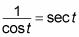##### Trigonometry Workbook For DummiesWhen the trig expression you're given begins with fractions, most of the time you have to add (or subtract) them to get things to simplify. Here's one example of a proof where doing just that gets the ball rolling. Say you have to find the lowest common denominator (LCD) to add the two fractions in order to simplify this expression: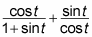With that as the beginning step, follow along:

1. In order to add these fractions, you must find the LCD of the two fractions.

The least common denominator is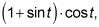so multiply the first term by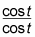and multiply the second term by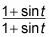You get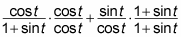2. Multiply or distribute in the numerators of the fractions.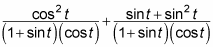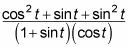4. Look for any trig identities and substitute.

You can rewrite the numerator as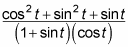which is equal to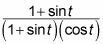because cos2 t + sin2 t = 1 (a Pythagorean identity).

5. Cancel or reduce the fraction.

After the top and the bottom are completely factored, you can cancel terms: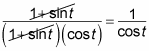6. Change any reciprocal trig functions.